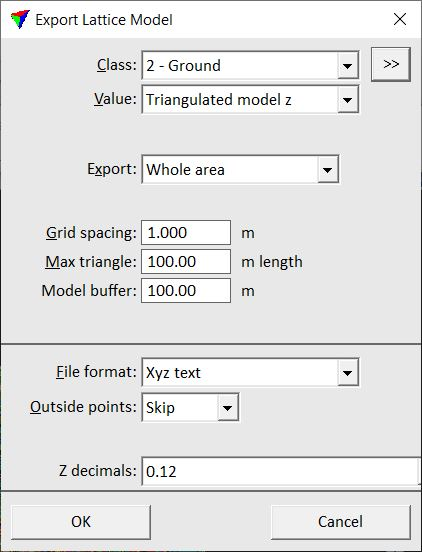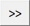# TerraScan User Guide

 Navigation: Tool Reference > TerraScan Window > Output pulldown menu Export lattice model

Export lattice model

Export lattice model command creates a grid file with uniform distances between points from one or more selected point classes. For each grid point, the lattice model file stores XY coordinates and one of the following value types:

elevation values

point count/density values

analytical values, such as average intensity, horizontal or vertical distance, surface roughness

There are several formats supported to store lattice models as raster or text files. The command requires the selection of at least one polygon that defines the lattice model boundary. If several polygons are selected, the software creates a separate lattice model file for each polygon. Text elements placed inside the polygon(s) can be used as file names for the lattice model files. A polygonal area is always expanded to a rectangular area of a lattice model. The cells outside the polygon are filled with a defined “outside” value or can be skipped from output in XYZ text files.

To export a lattice model:

1. (Optional) Draw rectangle(s) around areas from which you want to create lattice model(s). Place text elements inside the rectangles. Select rectangle(s) and text(s).

2. Select Export lattice model command from the Output pulldown menu.

This opens the Export Lattice Model dialog:3. Select settings and click OK.

This starts the lattice model file creation.

If File naming is set to Enter name for each, the Export lattice model dialog opens, a standard dialog for saving files.

4. Define a location and name for the lattice file and click Save.

Repeat step 4 for each lattice model.

SETTING

EFFECT

Class

Source class(es) for lattice model creation.Opens the Select classes dialog which contains the list of active classes in TerraScan. You can select multiple source classes from the list that are then used in the Class field.

Value

Value stored for each grid point of a lattice model:

Triangulated model z - elevation value calculated from a TIN model of the points in the source class(es).

Highest hit z - elevation value determined by the highest point in the source class(es).

Average hit z - elevation value calculated as average of points in the source class(es) falling inside the grid cell.

Lowest hit z - elevation value determined by the lowest point in the source class(es).

Closest hit z - elevation value determined by the point closest to the grid cell center in the source class(es).

Point count - amount of points falling inside the cell.

Point density - amount of points per squared master unit.

Average intensity - average intensity value of points in the source class(es) falling inside the grid cell.

Distance to point - average horizontal distance between a grid point and the two closest points in the source class(es).

Dz from ground - vertical distance between a grid point and a surface model created from the Ground class(es).

Surface roughness - difference of a grid point from a plane fitted to the closest points in the source class(es). Represents the local elevation variation of points in the source class(es).

Biggest distance - biggest distance value inside the grid cell. Requires that a distance value is computed for each point using the command for loaded points or corresponding macro action.

Average distance - average distance value of points inside the grid cell. Requires that a distance value is computed for each point using the command for loaded points or corresponding macro action.

Smallest distance - smallest distance value inside the grid cell. Requires that a distance value is computed for each point using the command for loaded points or corresponding macro action.

Ground

Source class(es) for creating a surface model. This is only active if Value is set to Dz from ground.Opens the Select classes dialog which contains the list of active classes in TerraScan. You can select multiple source classes from the list that are then used in the Ground field.

Export

Area to be exported into lattice model file(s):

Whole area - the area covered by the loaded points is exported into a single lattice model file.

Selected rectangle(s) - the area inside selected polygon(s) is exported into separate files.

Expand by

Distance by which a selected polygon is expanded for the model export. The area covered by the expanded polygon is included in a lattice model file. This is only active if Export is set to Selected rectangle(s).

Grid spacing

Defines the distance between grid points and thus, the resolution of the lattice model.

Max triangle

Maximum length of a triangle edge for TIN creation. Effects how big gaps are filled in the lattice model by interpolating grid point values from the TIN. This is only active if Value is set to Triangulated model z, or Distance to point.

Model buffer

Width of a buffer area around the actual model area that is considered for calculating grid point values. This is only active if Value is set to Triangulated model z, Distance to point, Dz from ground, or Surface roughness.

Fill gaps up to

Defines the size of gaps that are filled in the lattice model by deriving grid point values from closest points in the source class(es). This is only active if Value is set to Highest, Average, Lowest hit z, Average intensity, or Surface roughness.

File format

Format of the lattice model file: ArcInfo, GeoTIFF, Intergraph GRD, Raw, Surfer ASCII or binary, Xyz text.

Z unit

Unit of grid point values. Relevant for formats storing elevations as integers. This is only active if File format is set to GeoTIFF, Intergraph GRD, or Raw.

Outside points

Defines how the software handles grid cells that are not covered by points in the source class(es): Skip or Output. This is only active if File format is set to Xyz text.

Outside Z

Defines the value for grid cells that are inside the rectangular lattice model area but not covered by points in the source class(es). This is only active if File format is set to ArcInfo, GeoTIFF, Surfer ASCII and binary, Xyz text.

Z decimals

Determines the number of decimals stored for the grid point value. This is only active if File format is set to ArcInfo, Surfer ASCII, or Xyz text.

Create TFW files

If on, the software creates external georeference files for GeoTIFFs. This is only active if File format is set to GeoTIFF.

File naming

Defines how lattice model files are named:

Enter name for each - a name for each lattice model file has to be defined manually.

Selected text elements - selected text elements inside the lattice model area are used as file names.

This is only active if Export is set to Selected rectangle(s).

Directory

Directory for storing lattice model files. Click on the Browse button in order to select a folder in the Browse For Folder dialog. This is only active if File naming is set to Selected text elements.

Extension

Extension of lattice model files. There may be a specific extension required for a certain file format, such as .GRD for ArcInfo or .TIF for GeoTIFF. This is only active if File naming is set to Selected text elements.

 Lattice models can be produced in batch mode by using •Export lattice models command for project blocks.•Export lattice macro action.•Produce lattice models command in TerraModeler.

TerraScan User Guide   13.09.2022   © 2022 Terrasolid Ltd